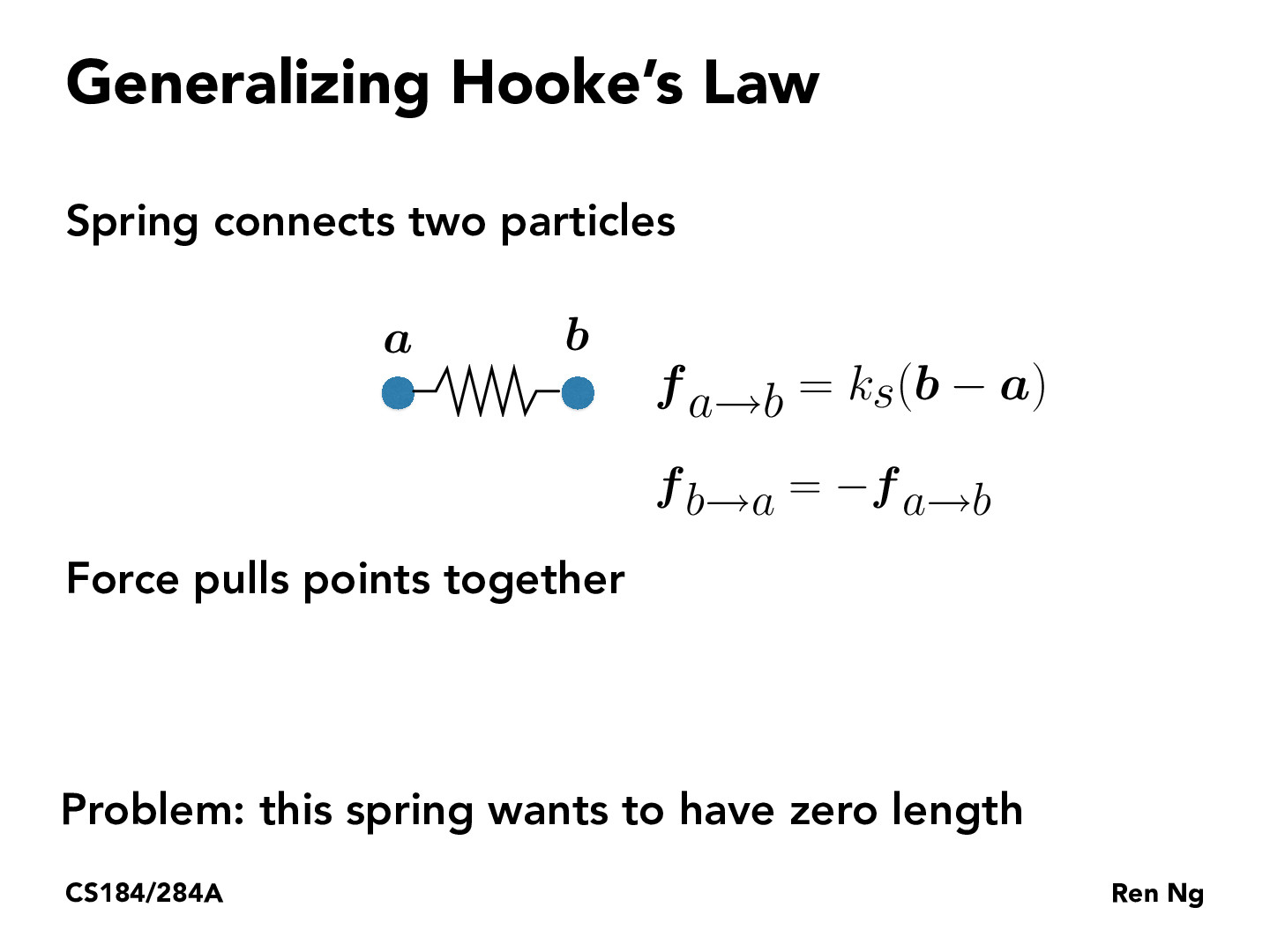You are viewing the course site for a past offering of this course. The current offering may be found here.Lecture 18: Physical Simulation (14)kevinliu64

I'm a bit confused as to what positive and negative forces mean. Does a negative force represent that two objects are repelling each other and the opposite for a positive force. Or am I misinterpreting the slide.serser11

The negative force just means the force would be applied to the opposite direction of the direction. The positive or negative in this case represent the directions in 2D case.chrisamez

Where do we get the problem that this spring wants to have zero length. Is f_a->b in this case a measurement of length?go-lauren

@chrisamez the forces acting on b and a are never going to be zero unless $b = a$ as we can see in the $f_{a \to b} = k_s (b-a)$ equation. Thus, the two forces will keep pulling b and a together until they are in the same spot i.e. the spring has zero length

You must be enrolled in the course to comment